# Thermal Analysis – Precision, Trueness, Accuracy and Errors

Trueness, accuracy and precision of results are very important in analytical measurements. A good understanding of the various sources of errors in measurement using an analytical method will help in optimizing different methods. Here we discuss these error sources in DSC (differential scanning calorimetry), TGA (thermogravimetric analysis), TMA (thermomechanical analysis) and DMA (dynamic mechanical analysis).

## Systematic and Random Errors of Measurement

Figure 1 shows that individual measurement values hover around a mean value and the difference between the mean value and true value is the systematic error of measurement or bias.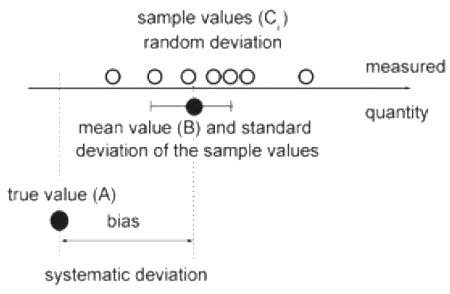Figure 1. The individual values of a measurement series (Ci) are scattered around a mean value (B) and show a certain standard deviation. The deviation of the mean value (B) from the true value (A) is the systematic error of measurement (or bias).

Systematic and random errors constitute the difference between a true value and an individual measurement. An example of systematic error in TGA is buoyancy. Systematic error can be rectified by carrying out a blank measurement. The result of the blank run is subtracted from the sample measurement. An example of random errors, also known as indeterminate errors, is the measurement of enthalpy of fusion of indium using DSC. Table 1 shows 100 DSC measurement values of the same sample.

Table 1. Determination of the enthalpy of fusion of indium (in J/g) by DSC; the same test specimen was measured one hundred times at 10 K/min.

 28.417 28.308 28.477 28.592 28.583 28.642 28.208 28.424 28.572 28.329 28.373 28.262 28.245 28.341 28.364 28.215 28.387 28.405 28.465 28.409 28.414 28.409 28.599 28.441 28.429 28.393 28.669 28.546 28.714 28.377 28.634 28.271 28.510 28.550 28.663 28.441 28.392 28.525 28.408 28.534 28.290 28.356 28.281 28.410 28.446 28.453 28.414 28.694 28.257 28.368 28.164 28.611 28.308 28.377 28.534 28.502 28.547 28.516 28.298 28.326 28.527 28.486 28.346 28.423 28.465 28.512 28.465 28.349 28.659 28.504 28.458 28.542 28.546 28.379 28.348 28.573 28.317 28.277 28.529 28.521 28.695 28.610 28.595 28.463 28.450 28.500 28.447 28.333 28.253 28.542 28.499 28.519 28.474 28.336 28.587 28.415 28.357 28.359 28.402 28.400

## Precision, Trueness and Accuracy

Accuracy is defined as the closeness of agreement between an individual value and a true value. Accurate results are free from systematic and random errors and accuracy also involves trueness and precision.

Precision is defined as the closeness of agreement between individual values. If the random error is small better precision can be achieved.

Trueness is described as the closeness of agreement between the mean value of a measurement series and the true value. The smaller the systematic error, the better the trueness of an analytical method.

Figure 2 shows a target board presentation for a set of measurement values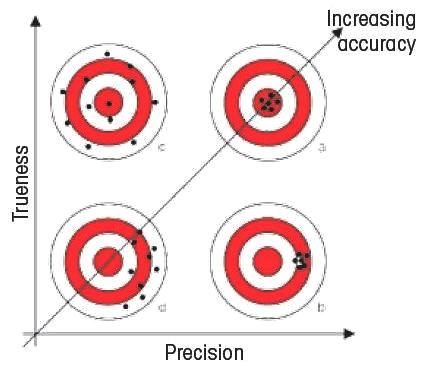Figure 2. The better the precision of an analytical procedure, the smaller is the random error of measurement of the individual values from the mean value. The trueness is independent of the precision. It describes the difference between the mean value and the true value (here the center of the target board).

## Important Sources of Measurement Errors

Several factors contribute to systematic and random measurement errors in analytical procedures. The significant ones are listed below:

• Influence of the procedure or method bias
• Instrumental influences
• Sampling and sample preparation
• Environmental influences
• Experimental parameters
• Evaluation methodology
• Time-dependent factors
• Shortcomings of the operator
• Gross errors

The above factors are detailed in the following sections.

## Influence of the Procedure

Different analytical procedures give different results. Figure 4 shows the measurement of Solid Fat Index using DSC and NMR.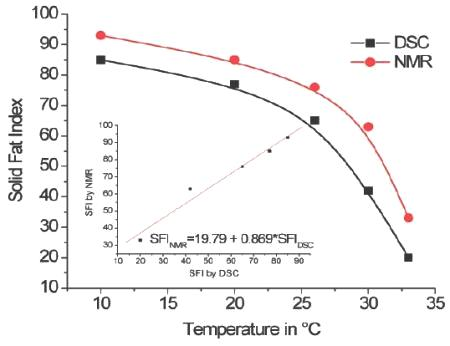Figure 3. Determination of the Solid Fat Index (SFI) by DSC and NMR.

## Instrumental Influences

A common cause for systematic errors in measurement is improper adjustment of the instrument used for the measurement.

## Sample Preparation

Sample selection and preparation is a major step in analytical measurements. Measurement errors can occur if the following factors are overlooked:

• Changes in the sample due to stress
• Change in the material properties over time, during storage or transport
• Instability or contamination of the sample
• Inaccurate sample weighing
• Inaccurate determination of the sample geometry

Figure 5 shows a good sampling plan, which follows the definition of consistent sampling process.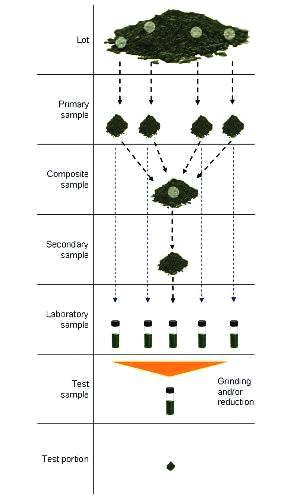Figure 4. Example of sampling operations (sampling plan).

## Environmental Influences

The measuring system should be insensitive to influences of its environment. In thermal analysis, the key factors affecting the measurement signal are given below:

• Pressure
• Temperature
• Vibrations
• Contamination

## Method Parameters and Evaluation

Conditions, such as sample mass, sample geometry, heating and cooling rate, atmosphere, temperature range, crucible, force, pressure, displacement and frequency, sampling and sample preparation and storage, in which a measurement is carried out must be monitored for each measurement.

## Time-Dependent Factors

Time is another key factor that affects measurement errors in a systematic way. For example, a sensor’s sensitivity can change over time.

## Shortcomings of the Operator

Individual abilities, practical skills, experience and theoretical knowledge of the operator also influence systematic and random errors of measurement.

## Gross Errors

Gross errors are a third type of errors mainly comprising the following:

• Wrongly transcribed results and measurement data
• Signs and rounding
• Errors in calculations
• Programming errors in computer programs
• Incorrect weighing or determination of sample geometry
• Mistaken identity of sample material or wrong reagent concentrations.

## Detection and Elimination of Measurement Errors

Systematic errors of measurement can be detected using comparative measurements. The three major comparative measurements are listed below:

• Deliberate change of the experimental parameters
• Use of a fundamentally different measuring method
• Interlaboratory studies (Round Robin studies)

## Conclusions

Accuracy involves both trueness and precision, whereas trueness is the closeness of agreement between the mean value and a true value and precision is the closeness of agreement between individual values. Trueness and precision are a measure of the systematic and random errors of measurement, respectively. The important causes of errors in measurement are influences of the procedure, instrumental influences, environmental influences, sampling and sample preparation, experimental parameters, shortcomings of the operator, evaluation methodology, and time-dependent factors. The accuracy of analytical methods can be enhanced by having a broad understanding of the measurement procedures and method development in a competent manner.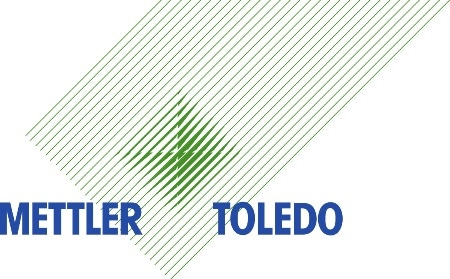This information has been sourced, reviewed and adapted from materials provided by Mettler Toledo - Thermal Analysis.

## Citations

• APA

Mettler Toledo - Thermal Analysis. (2020, January 07). Thermal Analysis – Precision, Trueness, Accuracy and Errors. AZoM. Retrieved on January 20, 2020 from https://www.azom.com/article.aspx?ArticleID=5744.

• MLA

Mettler Toledo - Thermal Analysis. "Thermal Analysis – Precision, Trueness, Accuracy and Errors". AZoM. 20 January 2020. <https://www.azom.com/article.aspx?ArticleID=5744>.

• Chicago

Mettler Toledo - Thermal Analysis. "Thermal Analysis – Precision, Trueness, Accuracy and Errors". AZoM. https://www.azom.com/article.aspx?ArticleID=5744. (accessed January 20, 2020).

• Harvard

Mettler Toledo - Thermal Analysis. 2020. Thermal Analysis – Precision, Trueness, Accuracy and Errors. AZoM, viewed 20 January 2020, https://www.azom.com/article.aspx?ArticleID=5744.

## Tell Us What You Think